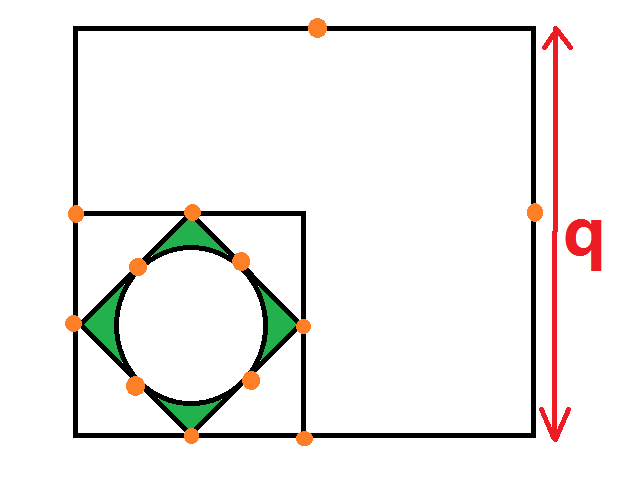# Maths Without Number.

Geometry Level 3The picture shows 3 quadrilateral and one circle. -All the quadrilateral are square. -All the orange dot are midpoint of its respective square. -The side of the largest square is q. -Given the area of shaded region (Green) is (3q^2)/[(3L^2) +4].

Use π = ­22/7­ , find the value of L.

×